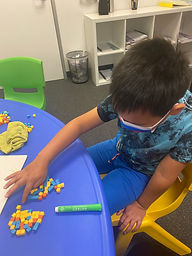## Ms. Briana

### Target 1​

###### Lesson Type:

Continuation

Number Operation

:

Computation

Divide within 100.

###### 1:

Understand that dividing numbers makes numbers smaller.

###### 2:

Understand that division represents taking a quantity and determining how many equal groups of objects can be made.

###### 3:

Understand there are multiple ways to divide a multi-digit number.

###### 4:

Understand that division equations can be written both vertically and horizontally and division can be indicated using multiple symbols (i.e., ÷, ⟌).

3rd

###### Vocabulary:

Dividing, Quantity, Repeated Subtraction

Activities:

Students used dice to division equations.

Students used centimeter cubes as a visual representation of groups with equal quantities.

Students worked together on the large whiteboard to practice standard form.### Home Exploration

###### Guiding Questions:## Absent Students:

Nova

### Target 2

:

###### 1:

Understand and explain what a fraction is.

###### 2:

Identify the number of shaded or unshaded parts as the numerator of a fraction.

###### 3:

Identify the number of equal parts as the denominator of a fraction.

###### 4:

Compose a written fraction to describe a visual or a visual to describe a written fraction.

3rd

###### Vocabulary:

Fraction, Quantity, Divided, Denominator and Numerator

Activities:

Students made and used "pizzas" to represent cutting a whole into peices.

Students used total number of slices as the visual for the denominator.

Students rolled dice to determine the number remaining or being eaten as a visual for the numberator.### Home Exploration

###### Guiding Questions:### Target 3

:

###### Vocabulary:

Activities:### Home Exploration